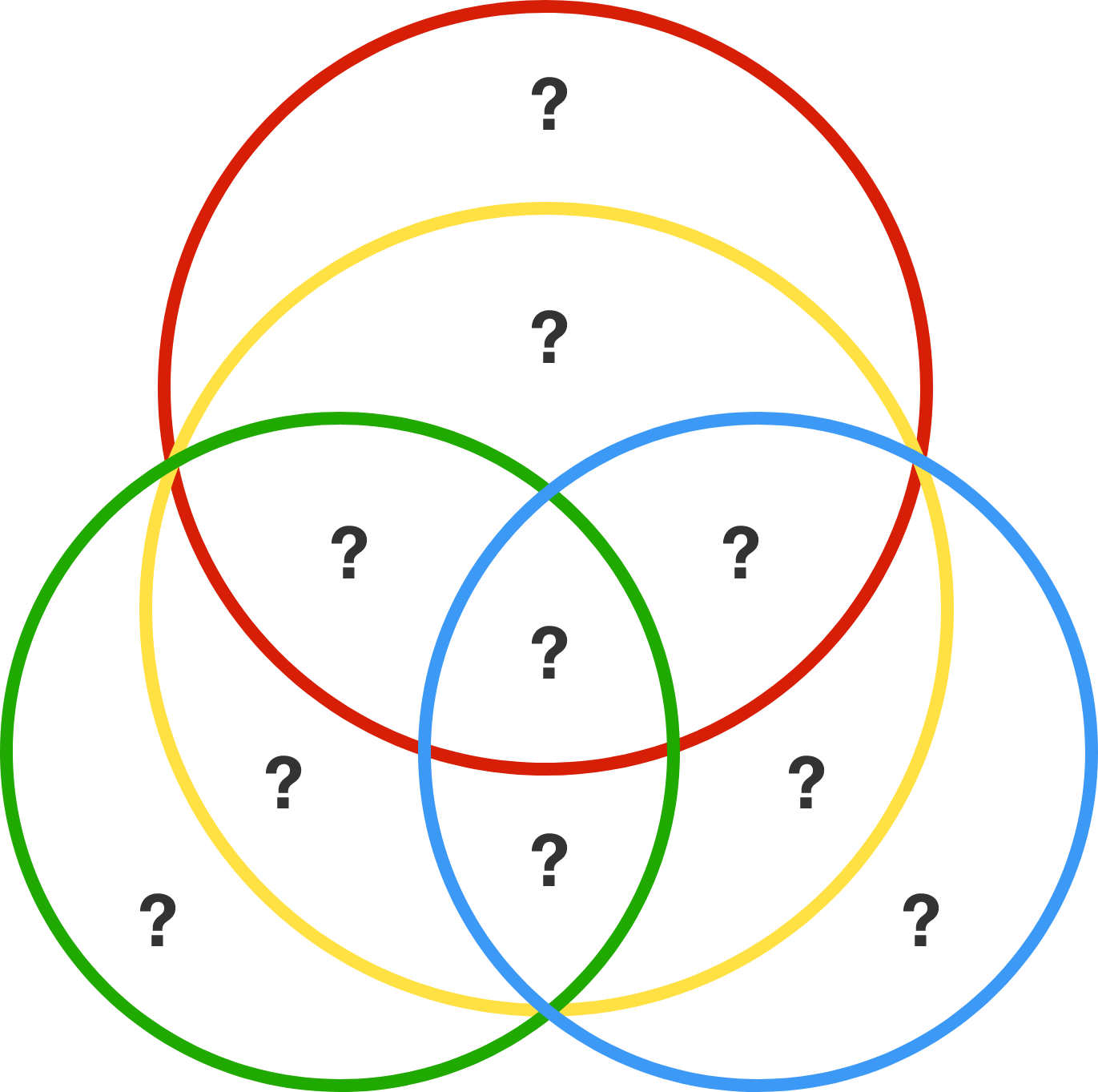# Numbers in circles

Algebra Level 2

4 circles overlap and divide one another into a total of 10 parts, as indicated by the 10 question marks in the diagram. Replace each question mark with a distinct digit 0 to 9, such that the sum of the digits in each circle is equal to the same value $x$.

Find the maximum possible value of $x$.×

Problem Loading...

Note Loading...

Set Loading...